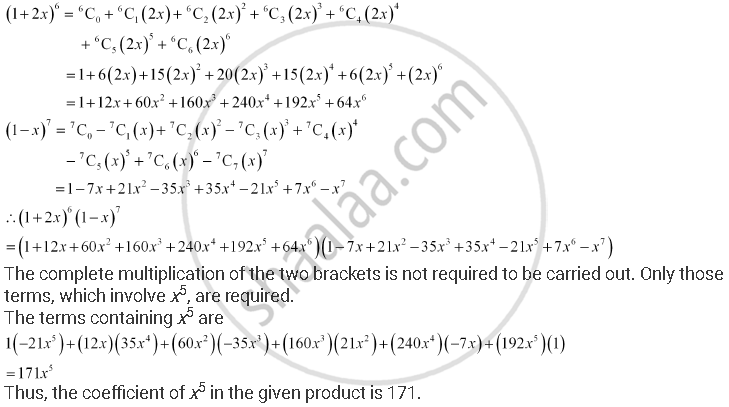CBSE (Arts) Class 11CBSE
Share
Notifications

View all notifications
Books Shortlist
Your shortlist is empty

# Find the Coefficient of X5 in the Product (1 + 2x)6 (1 – X)7 Using Binomial Theorem. - CBSE (Arts) Class 11 - Mathematics

Login
Create free account

Forgot password?
ConceptBinomial Theorem for Positive Integral Indices

#### Question

Find the coefficient of x5 in the product (1 + 2x)6 (1 – x)7 using binomial theorem.

#### Solution

Using Binomial Theorem, the expressions, (1 + 2x)6 and (1 – x)7, can be expanded asIs there an error in this question or solution?

#### APPEARS IN

Solution Find the Coefficient of X5 in the Product (1 + 2x)6 (1 – X)7 Using Binomial Theorem. Concept: Binomial Theorem for Positive Integral Indices.
S